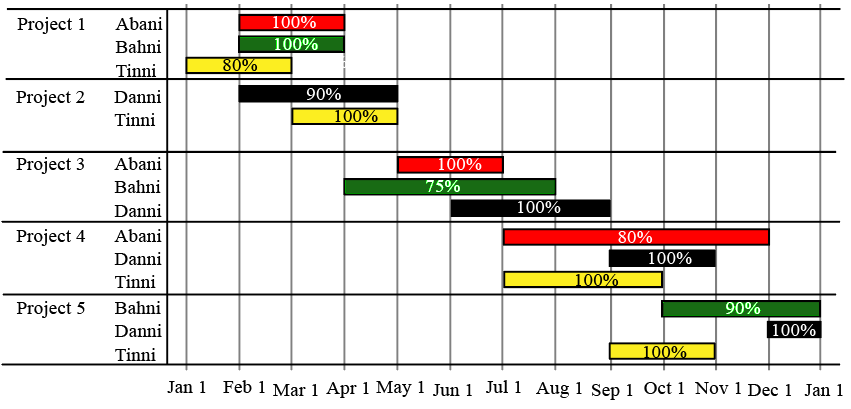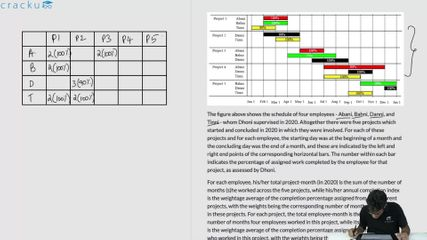### CAT 2021 Question Paper (Slot 3) Question 32

InstructionsThe figure above shows the schedule of four employees - Abani, Bahni, Danni, and Tinni - whom Dhoni supervised in 2020. Altogether there were five projects which started and concluded in 2020 in which they were involved. For each of these projects and for each employee, the starting day was at the beginning of a month and the concluding day was the end of a month, and these are indicated by the left and right end points of the corresponding horizontal bars. The number within each bar indicates the percentage of assigned work completed by the employee for that project, as assessed by Dhoni.

For each employee, his/her total project-month (in 2020) is the sum of the number of months (s)he worked across the five projects, while his/her annual completion index is the weightage average of the completion percentage assigned from the different projects, with the weights being the corresponding number of months (s)he worked in these projects. For each project, the total employee-month is the sum of the number of months four employees worked in this project, while its completion index is the weightage average of the completion percentage assigned for the employees who worked in this project, with the weights being the corresponding number of months they worked in this project.

Question 32

# The list of employees in decreasing order of annual completion index is:

Solution

The annual  completion index for different people is :

The weightage average of the completion percentage assigned from the different projects, with the weights being the corresponding number of months (s)he worked in these projects.

For Abani :

$$\frac{\left(\left(100\cdot2\right)+\left(100\cdot2\right)+\left(80\cdot5\right)\right)}{2+2+5}=\ \frac{800}{9}$$

For Bahni :

$$\frac{\left(\left(100\cdot2\right)+\left(75\cdot4\right)+\left(90\cdot3\right)\right)}{2+3+4}=\ \frac{770}{9}$$

For Danni :

$$\frac{\left(\left(90\cdot3\right)+\left(100\cdot3\right)+\left(100\cdot2\right)+\left(100\cdot1\right)\right)}{2+3+2+1}=\ \frac{870}{9}$$

For Tinni :

$$\frac{\left(\left(80\cdot2\right)+\left(100\cdot2\right)+\left(100\cdot3\right)+\left(100\cdot2\right)\right)}{2+2+3+2}=\ \frac{860}{9}$$

The descending order for the four people is :

Danni, Tinni, Abani, Bahni.

### View Video Solution• All Quant CAT Formulas and shortcuts PDF
• 30+ CAT previous papers with solutions PDF